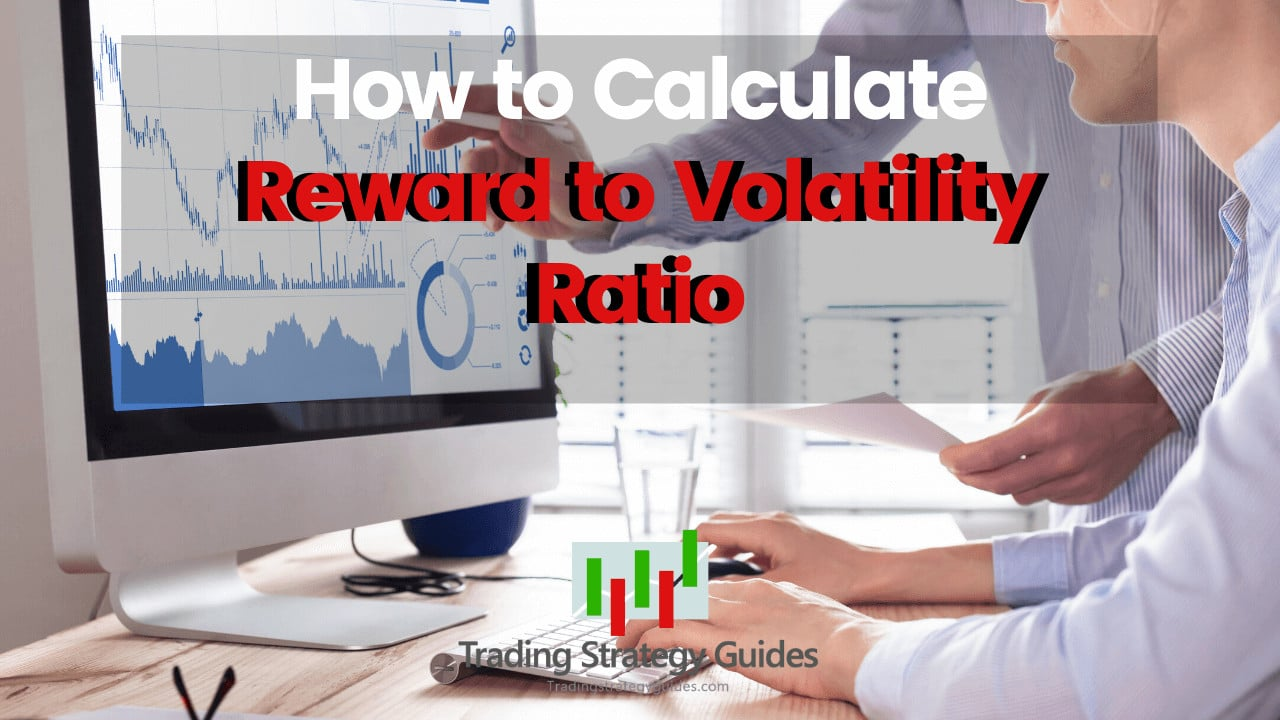Learn how to calculate reward to volatility ratio with this step-by-step guide. The reward to volatility ratio, also known as the Sharpe ratio is one of the most important risk metrics to assess an investment. This guide will explain the Sharpe ratio, how to use the reward to volatility ratio calculator and its importance in minimizing risk in your portfolio.

If this is your first time on our website, our team at Trading Strategy Guides welcomes you. Make sure you hit the subscribe button, so you get your Free Trading Strategy every week directly into your email box.

Whenever investment performance is being discussed the most commonly used risk metric on Wall Street is the Sharpe ratio. We can’t judge effectively the overall performance of a portfolio exclusively on total return. That’s where these risk-adjusted metrics come in handy.

Many times, excessive returns can be the result of taking excessive risk, rather than genuine investing skills. For example, investing in cryptocurrencies was synonymous with excessive risk and that lead to record high returns.

While volatility creates risk, it also creates opportunities for strong returns. By understanding how to exploit volatility, you can become a successful trader.

Do you want to know what the best cryptocurrency to invest in for 2019 is?

Make sure you see: Best Cryptocurrency to Invest in 2020 – Our Top 5 Picks.

Now, if you don’t possess enough financial knowledge to understand the jargon, don’t worry, we’ve got your back. Moving forward, we’re going to discuss what is the Sharpe Ratio, how it’s useful, and what are some of the pitfalls.

# What is Reward to Volatility Ratio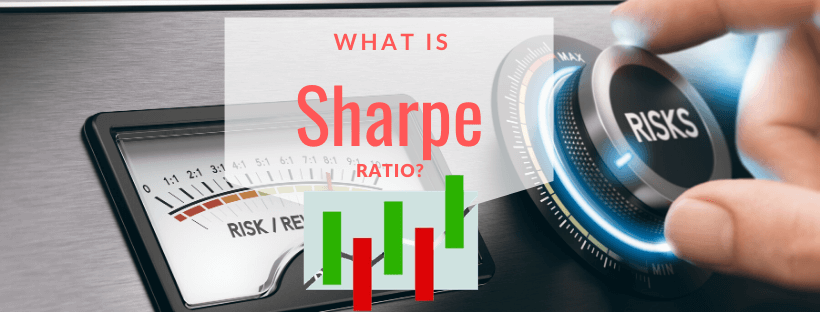In finance, the reward to volatility ratio, also commonly known as the Sharpe Ratio, is a measure of risk-adjusted return for a stock or a stock portfolio. It’s often used to measure the performance of an investment relative to the risk taken to generate that return.

Note* moving forward, we’re going to use both reward to volatility ratio and Sharpe ratio interchangeably.

In simple words, the reward to volatility ratio helps investors assess the return of an investment compared to its risk.

Originally it was named after American economist and Nobel laureate, William Sharpe, and over the course of the years, the Sharpe Ratio has undergone some alterations.

Nowadays, we can distinguish two variations of the Sharpe Ratio:

1. Sortino Ratio
2. Treynor Ratio

The Treynor ratio reveals whether or not the fund manager is remunerated for the extra risk they take, above the inherent market risk. On the other hand, the Sortino Ratio focuses on removing the impact of increasing price movements on the standard deviation.

What is a good Sharpe ratio?

Let’s see the Sharpe ratio interpretation:

• Sharpe ratio below 1 is a bad investment.
• Sharpe ratio between 1 – 1.99 is a good investment.
• Sharpe ratio between 2 – 2.99 is a great investment.
• Sharpe ratio greater than 3 is an amazing investment.

The basic principle is that a higher reward to volatility ratio is better.

The higher the Sharpe ratio is, the better.

In essence, a high Sharpe ratio is telling us that a portfolio generated high returns without taking an excessive risk to achieve those types of returns.

Let’s now jump and see the math being the Sharpe Ratio and learn how to calculate reward to volatility ratio.

# How to Calculate Reward to Volatility Ratio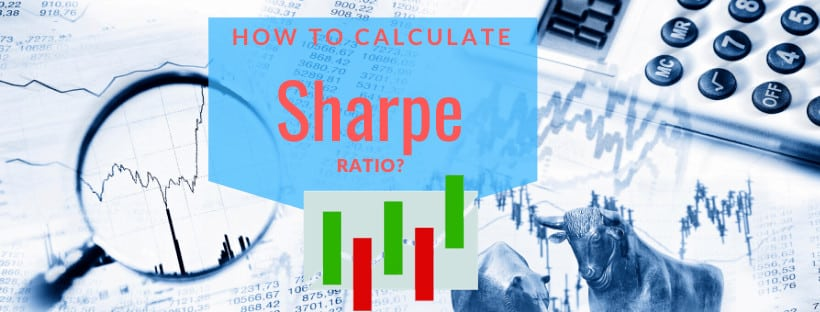The Sharpe Ratio formula:

Sharpe Ratio=((Rx-Rt))/(StdDev Rx)

Where:

• Rx = Expected portfolio return
• Rf = Risk-free rate of return
• StdDev Rx = Standard deviation of portfolio return/volatility

The risk-free rate is usually the return on a benchmark bond like a 10 year Treasury bond.

While it’s good to know the math behind the Sharpe Ratio, there is no need to waste your time in doing the math.

You can simply use for free a reward to volatility ratio calculator.

Simply google Sharpe Ratio calculator and you’ll have at your disposal different options.

Let’s see how the Sharpe Ratio, Treynor Ratio, and Sortino Ratio differ from one another:

The Treynor ratio formula is the following:

Treynor Ratio=((Portfolio return-Risk Free Rate))/(Portfolio Beta)

The only difference between the Sharpe ratio and the Treynor ratio is that we use something known as the Portfolio Beta instead of the standard deviation.

The Sortino ratio formula is the following:

Sortino Ratio=((Portfolio return-Requiered Return))/(Distribution of Returns that are below target return )

The Sortino ratio uses in its calculation the required return instead of the risk-free rate and the distribution of returns that are below the required return.

Here is a simple Sharpe Ratio example, to help you understand how to calculate the Sharpe ratio.

See below:

# Example of Using the Sharpe Ratio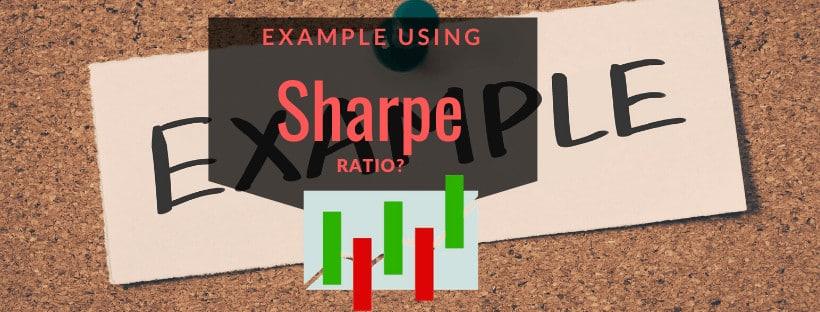For example, let’s assume you want to diversify your current investment portfolio that is split between stocks and bonds.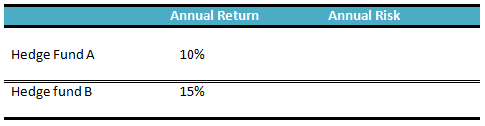You have Hedge Fund A and Hedge Fund B.

Both of these hedge funds have posted double-digit annual return.

This is great!

At first glance, Hedge Fund B generated more profits.

Between 15% annual return and 10% annual return, which investment portfolio would you choose?

It’s no shame to pick the portfolio that generated the most profits. After all, we’re in the business of making money. The more ROI (return of investment) we generate the better!

Most people will pick the portfolio with the highest annual return.

But, what if we add into the mix the risk involved to generate this type of return.

Here is the annual risk for each Hedge Fund: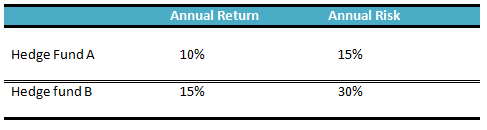Now, we get a different picture and perspective of those annual returns.

The annual risk of Hedge Fund A is 15%, but Hedge Fund B has an annual risk of 30%.

Which investment portfolio you want now?

Hmmm….

Maybe you’re not sure.

But, here is where the Sharpe Ratio comes in.

Using the reward to volatility ratio we can calculate the risk/return trade-off.

The Sharpe ratio will put those two components, risk, and return together and show you which investment portfolio looks better.

The only missing component for our Sharpe ratio example is the risk-free rate.

Once we have that missing link we can input these numbers into the reward to volatility ratio calculator or use the Sharpe Ratio formula.

Which, for simplicity, we’re going to assume that the 10-year Treasury bond is 1%.

We’re going to marry all these numbers together and see which Hedge Fund manager is more skilled in generating risk-adjusted returns.

Before we learn how to calculate reward to volatility ratio, let’s remember again the Sharpe Ratio formula:

Sharpe Ratio=((Rx-Rt))/(StdDev Rx)

Using the risk metrics mentioned in this section, we would have a calculation mechanically that will generate the following:

• Hedge Fund A has an annualized Sharpe Ratio equal to 0.6
• Hedge Fund B has an annualized Sharpe Ratio equal to 0.46

Here is the Sharpe Ratio interpretation:

One way to look at it is a high Sharpe ratio is better than a low Sharpe Ratio.

In this case Hedge Fund A portfolio is the winner.

The Sharpe ratio is telling us that Hedge Fund manager A is squeezing out more return per unit of risk.

Now, Hedge Fund manager B has two options if he wants to increase his Sharpe ratio.

First, he can increase his returns.

Or, secondly, he can obtain higher returns if he can reduce the up and down swings in his account balance. In other words, by reducing the annual risk, he can generate a better Sharpe ratio.

Let’s see some of the advantages of using the Sharpe ratio.

See below:

# Why Use Sharpe Ratio VolatilityProfessional hedge fund managers can’t speak about the annual return without also mentioning some of the risk-adjusted metrics like the Sharpe Ratio.

You know why?

Because annual returns on their own are meaningless on Wall Street. Read about other popular Hedge Fund Strategies here.

When retail traders talk about investment results, it’s very often that the only number we see is the X percentage rate of return.

Maybe they have made a 100% return on their investment in the past year.

On the surface, it sounds good and it’s definitely a good performance.

Traders get overly excited when they see these types of annual returns.

However, the issue is that we have no idea how those triple-digit returns were achieved.

Or, the trader, achieved those results due to excessive risk?

Maybe, the trader got lucky because we’re in a secular bull market and it happened to work out well for him.

One way to find out if the results are simply the result of luck or genuine edge in the market is to look at risk metrics such as the Sharpe Ratio.

The reward to volatility ratio will tell us the probability to expect the same type of results to continue going forward. The Sharpe Ratio will reveal if the account balance volatility is too high or if the drawdown is to large.

By quantifying the risk-adjusted return we can pinpoint how a trader or a hedge fund manager generated his profits.

Now that we’ve examined the advantages of using the Sharpe ratio, let’s see some of the Sharpe ratio limitations.

See below:

# Disadvantages of Using the Sharpe RatioIt’s well notable that the Sharpe ratio can oversimplify risk.

Hedge Fund managers know that traders watch these ratios, so to some degree, they can manipulate the Sharpe ratio.

We want to only highlight three potential problems in using the reward to volatility ratio to measure trading performance:

• Sharpe ratio focuses on price and risk predominantly. A fund manager can fool you by taking liquidity or default risk that may not show up in the Sharpe ratio calculation.
• If a Fund Manager tries to boost returns using derivatives that often won’t show up in the Sharpe ratio calculation either.
• The Sharpe ratio is based on historical return so it looks backward. There is no guarantee that the Hedge fund manager will be able to generate the same type of positive returns.

In today’s volatile market environment, it’s not uncommon to see hedge fund managers boosting returns by all means available.

# Final Words – Reward to Volatility Ratio Calculator

The reward to volatility ratio is a great tool to judge if the outperformance of a portfolio’s return is the result of a hedge fund manager’s skill or just the result of taking additional risk. By using a reward to volatility calculator an investor can assess a fund’s total performance on a risk-adjusted basis. The Sharpe ratio can gauge the quality and the impact of adding or subtracting a new asset or asset class from a portfolio. Also, be sure to check out our free Forex Position Size Calculator.

If you want to get a deeper understanding of a portfolio performance or stock performance you should take into consideration the risk element. Simply, learn how to calculate reward to volatility ratio if you want to mix together the risk and reward when considering an investment choice.